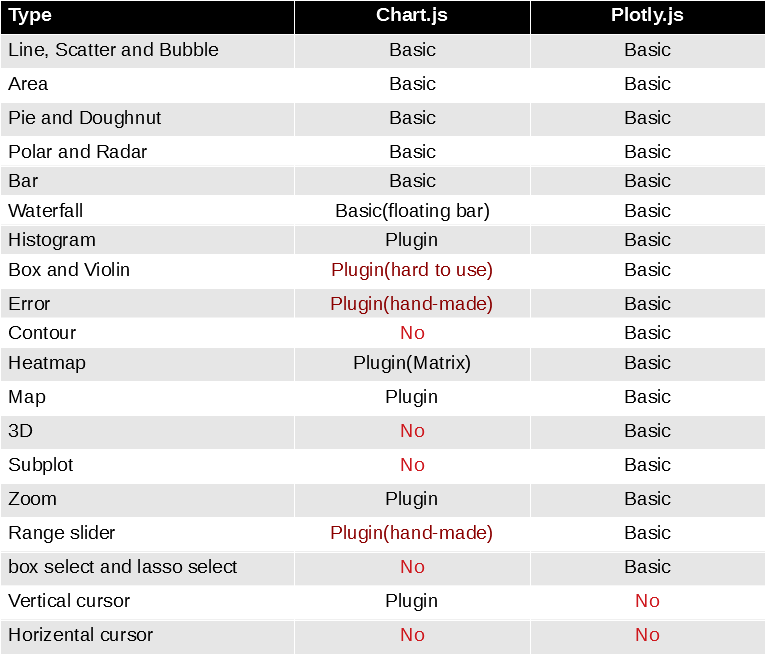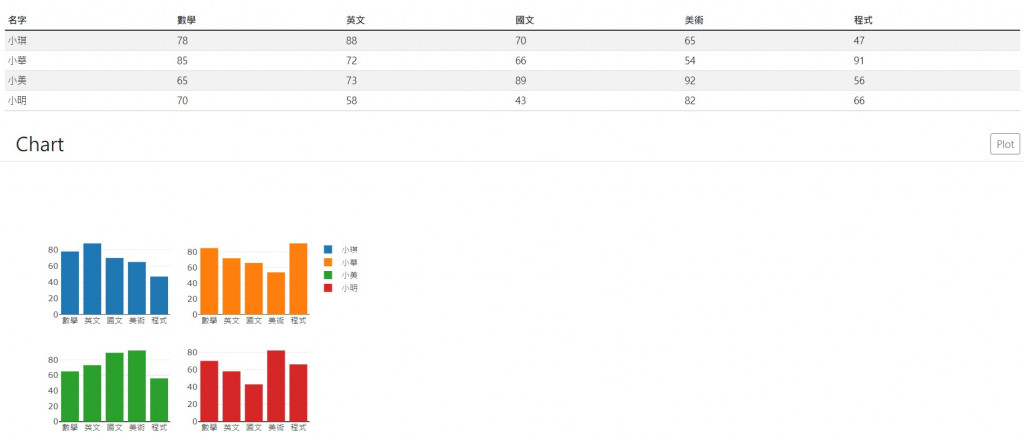#DAY 19
0

## Day19有比較有傷害

• plotly.js``````  function plot() {
const data = array.slice(1).map((item, index) => {
temp_x = 'x';
if (index > 0) {
temp_x = temp_x + (index + 1);
}
temp_y = 'y';
if (index > 0) {
temp_y = temp_y + (index + 1);
}
return {
x: array.slice(1),
y: item.slice(1),
name: item,
type:'bar',
xaxis: temp_x,
yaxis: temp_y,
};
});
const layout = {
grid: {rows: 2, columns: 2, pattern: 'independent'},
};
Plotly.newPlot("MyChart", data, layout);
}
``````